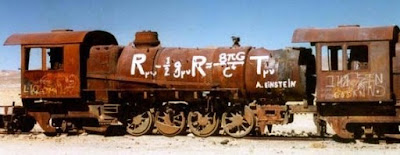## Sunday, January 25, 2009

### Einstein train...rusted and retiredMost unusual. The photo would make good for wallpaper or even a poster.

From Physics Central:

A train that describes how one might travel through spacetime was discovered in the Bolivian desert by a physics teacher. The old locomotive itself must have pushed the boundaries of space and time due to its mysterious location in the desert and its signs of old age. The unique feature of the train is the expression painted on the engine and signed by A. Einstein. Whether Albert Einstein painted or used this train himself remains unknown. But the significance of the painted expression goes beyond historical accuracy. While everyone can recite Einstein’s famous equation E=mc2, few know that he went even further to describe the relationship between mass and the shape of spacetime. This equation not only answers the question of how gravity works, but it also describes black holes, gravity waves, bending of light, planetary motion, and the shape of the universe. For instance, the left side of the equation with the big R’s represents the curvature and shape of spacetime and hence the path that an object follow. The right side of the equation with the big T represents the mass and energy density of a star or planet. Putting the two sides of the equation together says that a massive object like a star or planet will curve the surrounding spacetime. As a result this also curves the path of other objects moving past such as comets or satellites. Unfortunately the mathematics involved in this equation is quite advanced. In fact it was said that only a handful of people in the world understood it when Einstein proposed his theory of relativity. This kind of mathematics is called tensor calculus which is like regular calculus but in multiple dimensions. With such an intimidating mathematical equation staring at you from a train, one can take comfort in recognizing some of the symbols such as 8 and pi. Pi is a familiar term from geometry and interestingly enough that fraction helps to convert the mass energy density into the geometry of space and time.

Coincidentally, Einstein often used the example of observers traveling on a train to describe his theory of relativity. The observer on this particular day was Monica Witt, a physics teacher at Friends Seminary in New York City, who discovered this fascinating artifact while hiking through the Bolivian desert.